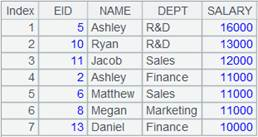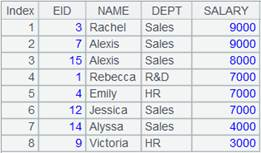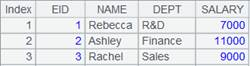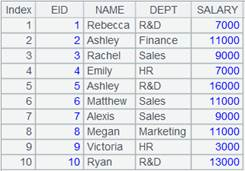# groupx()

## ch.groupx()

ch.groupx(x:F,…;y:G…)

 @n x取值为分组序号，可直接定位

 ch 管道 x 分组表达式 F 结果序表的字段名 y ch的聚合函数，仅支持sum/count/max/min/top/iterate，使用iterate(x,a;Gi,…)时不能有参数Gi。 G 结果序表中的汇总字段名

 A 1 =demo.cursor("select * from EMPLOYEE ") 2 =channel() 创建管道 3 =channel() 创建管道 4 =A1.push(A2,A3) 将游标A1中的数据推送到管道A2和A3，此时数据不会立即被推送到管道。 5 =A2.groupx(DEPT:dept;sum(SALARY):TotalSalary) 指定字段DEPT分组，并按照指定字段排序 6 =A3.groupx@n(if(GENDER=="F",1,2):SubGroups;sum(SALARY):TotalSalary) x取值为分组序号，GENDER等于“F”的分到第一组，否则分到第二组，并且每个组聚合 7 =A1.select(month(BIRTHDAY)==2) 8 =A7.fetch() 游标A7添加了计算。 9 =A2.result() 结果返回成游标 10 =A3.result() 结果返回成游标

## cs.groupx()

cs.groupx(x:F,…;y:G…;n)

 @n x取值为分组序号，可直接定位 @u 结果集不再按x排序；与@n互斥 @g 参数n解释为分段表达式，先根据表达式n分段，分段后再分组排序 @b 返回的游标中只有聚合数据，没有分组列数据

 cs 游标记录 x 分组表达式 F 结果字段名 y cs的聚合函数，仅支持sum/count/max/min/top/iterate，使用iterate(x,a;Gi,…)时不能有参数Gi。 G 汇总字段名 n 缓冲区行数，运算过程中如果分组数达到n，则把分组结果写入临时文件中；n<1时缓冲区用缺省值的n倍；缺省值由集算器自动计算

 A 1 =demo.cursor("select * from SCORES where CLASS='Class one'") 2 =A1.groupx(STUDENTID:ID;sum(SCORE):Scores).fetch()3 =demo.cursor("select * from FAMILY") 4 =A3.groupx@n(if(GENDER=="Male",1,2):ID;sum(AGE):TotalAge).fetch()x取值为分组序号，GENDER等于“Male”的分到第一组，否则分到第二组，并且每个组聚合 5 =demo.cursor("select * from EMPLOYEE") 6 =A5.groupx@u(STATE:State;count(STATE):TotalScore).fetch()结果集无序 7 =demo.cursor("select * from EMPLOYEE where EID <=20") 8 =A7.groupx@g(GENDER;min(SALARY);EID>=10).fetch()以EID>=10为分段条件，分段后计算min(SALARY)，后两行为EID>=10的分组结果 9 =A1.groupx(STUDENTID:ID;sum(SCORE):Scores;3).fetch() 运算过程中分组数达到3，则把分组结果写入临时文件中 10 =demo.cursor("select * from scores where class = 'Class one'") 返回游标 11 =A11.groupx@b(STUDENTID:StudentID;sum(SCORE):TotalScore) 对A18游标中的序表数据进行分组聚合计算，计算后结果中只有聚合数据无分组数据，返回结果为游标 12 =A11.fetch() 取出A11游标中的数据：## cs.groupx()

cs.groupx(x:F,…;y:G…;n)

 cs 集群游标 x 分组表达式 F 结果字段名 y cs的聚合函数，仅支持sum/count/max/min/top/avg/iterate，使用iterate(x,a;Gi,…)时不能有参数Gi。 G 汇总字段名 n 缓冲区行数，运算过程中如果分组数达到n，则把分组结果写入临时文件中；n<1时缓冲区用缺省值的n倍；缺省值由集算器自动计算

 A 1 [192.168.31.72:8281, 192.168.31.72:8291] 2 =file("orderpart.ctx":, A1) 打开集群文件 3 =A2.open() 打开集群组表 4 =A3.cursor() 返回集群游标 5 =A4.groupx(EID:ID;count(~):IdCount) 按EID对A7分组计数，返回集群游标 6 =A5.fetch() 7 =A3.cursor() 8 =A7.groupx(if(EID==4,1,2):ID;count(~):IdCount) EID等于4的分到第一组，否则分到第二组，并计算每组EID个数 9 =A8.fetch() 10 =A7.groupx(if(EID<=10):ID;count(~):IdCount;2) 运算过程中分组数达到2，则把分组结果写入临时文件中